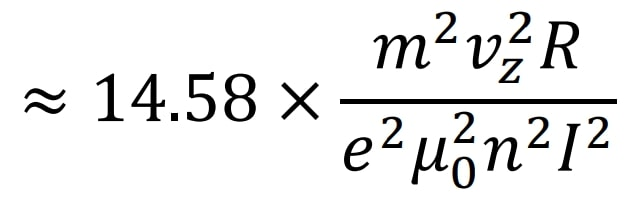# Electron Beam in Magnetic LensYou may derive that for a solenoid with a finite length, the magnetic field along its axis equals to

B = \frac{\mu_0nI}{2}(\cos\theta_1+\cos\theta_2),

where \theta_1 and \theta_2 are as denoted in the pic below.

In other words,

\vec B(z)=\frac{\mu_0nI}{2}\left(\left(1+\left(\frac{R}{z+L/2}\right)^2\right)^{-1/2}+\left(1+\left(\frac{R}{z-L/2}\right)^2\right)^{-1/2}\right)\hat z.

Also, using \displaystyle\oint\vec B\cdot d\vec S=0 for a small cylinder of thickness dz and radius r, you may find that

B_r = \frac{r}{2}\frac{dB(z)}{dz}.

I think this info would be helpful for you to tackle this problem.

3 лайка

Thank you, I’ll try again.